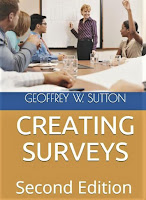## Monday, February 26, 2018

### Independent Samples t-test

Independent Samples t-test

Researchers use the independent samples t-test to find out if there is a significant difference between two sets of data. In the behavioral sciences, the data are often two sets of scores on tests or survey items.

Significance can mean a lot of different things. In behavioral science, it is common to think of significance as a frequently occurring, and thus reliable, difference. Sometimes the language of statistics can be confusing. The independent sample t-test evaluates the differences between the arithmetic means of the two groups of scores, and assumes the scores are normally distributed.

Usually, a difference needs to be at least large enough that a score difference as large, or larger than the one obtained, occurs only 5% of the time by chance.

The calculations are usually done in spreadsheets like Excel or Google Sheets or in a program like SPSS

You will find the following text in Chapter 16 of Creating Surveys. I also cover t-tests in Statistics for Counselors.

Creating Surveys

Create better surveys for work and school

The statistical procedure results in a t-value and a p value. The larger the t-value, the more likely it is that the difference between the group means is significant. The p-value represents the probability that the t-value of the size obtained did not occur by chance. As in many cases, the p-value is reported based on a 95% probability that the t-value did not occur by chance. The p-value is usually reported by considering the likelihood that it occurs by chance greater or less than 5% of the time where 5% is written as .05. If the probability is less than .05 that a t-value as large or larger than the one obtained did not occur by chance, the researcher would report the results as p < .05. If the t-value was too small to meet the level needed for significance, the researcher would just report the finding as not significant (p > .05).

I and my colleagues have studied forgiveness. Suppose we found that people who attended a forgiveness seminar obtained higher forgiveness scores compared to those who did not attend.
Here’s how we might write the results (Chapter 16, Creating Surveys).

We found that people who did attended the forgiveness seminar were significantly more forgiving (M = 39, SD = 5) than were people who did not attend the seminar (M = 30, SD = 5), t (98) = 6.75, p < .05.

Applied Statistics: Concepts for Counselors

Also, here is an online calculator where you can enter two sets of values and obtain a t-test result.
EasyCalculation.com

My Page    www.suttong.com

My Books   AMAZON

ResearchGate   Geoffrey W Sutton   (PhD)